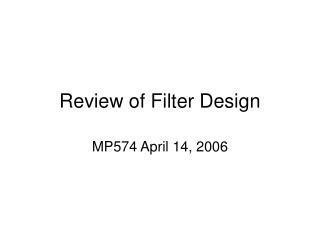DownloadDownload PresentationReview of Filter Design

# Review of Filter Design

Download Presentation## Review of Filter Design

- - - - - - - - - - - - - - - - - - - - - - - - - - - E N D - - - - - - - - - - - - - - - - - - - - - - - - - - -
##### Presentation Transcript

1. Review of Filter Design MP574 April 14, 2006

2. Difference Equation Implementation • Shift theorem of z-transform:

3. Difference Equation Implementation • Shift theorem of z-transform: FIR

4. Filter Design: IIR vs. FIR • Rules of thumb: • Use IIR when the only important requirements are sharp cutoff and high throughput • Use FIR in any application where linear phase is required

5. FIR Filter Design • Phase Delay • Tp = -q(w)/w • Tg= -dq(w)/dw • 4 Types of Linear Phase • Positive symmetry, odd number of coefficients • Tp = (N-1)/2 Tsample • Positive symmetry, even number of coefficients • Tp = (N/2-1/2)Tsample • Zero at w = p • Negative, odd • Negative, even • Tg = (N-1-p)/2 Tsample • p/2 phase shift • Zero at w = 0

6. Design Steps • Filter specification • dp peak passband deviation (or ripple) • ds stopband deviation • fp passband edge frequency • fs stopband edge frequency • Fs sampling frequency • Coefficient calculation • Coefficients of H(z) • Realization • Implementation

7. Matlab: fdatool

8. IIR: Chebyshev Type II Order 10

9. IIR: Chebyshev Type II Order 10

10. FIR: Hamming Window Order 30

11. FIR: Hamming Window Order 30

12. Sptool Import

13. Sptool Apply Filter

14. Summary of Filter Design • Range of polynomial forms of different orders • IIR converges to specifications more rapidly • Non-linear phase • Can be unstable in implementation • FIR most commonly used for medical implementations • Linear phase: signal preserved without distortion • More constraints require higher order fits • Stable and robust to quantization errors • Matlab tools for filter design • Take some time to familiarize yourself with them

15. Extension to 2D • Parks-McClellan Transformation • Step 1: Translate specifications of H(w1,w2) to H(w) • Step 2: Design 1D filter H(w) • Step 3: Map to 2D frequency space cosw = - ½ + ½ cosw1 + ½ cosw2 + ½ cosw1 cosw2 = T(w1,w2) - Step 4: determine h(n1,n2) by 2D FT.

16. Hamming Window Example

17. Hamming Window Example >> w1 = -pi:0.01:pi; >> w2 = -pi:0.01:pi; >> [W1,W2] = meshgrid(w1,w2); >> H_2d = 0.54+0.46.*(-0.5+0.5.*cos(W1)+0.5.*cos(W2)+0.5.*cos(W1).*cos(W2)); >>figure;mesh(H_2d) filter2()

18. 2D FIR Filter Design, Parks-McClellan

19. “firdemo”

20. Improvement After Affine Registration to Mask Frame

21. T1 fitting There are two slices only one of which contains the lesion (arrow)